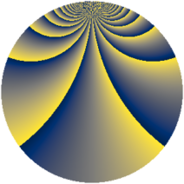# Properties

 Label 3549.2.drLevel $3549$ Weight $2$ Character orbit 3549.dr Rep. character $\chi_{3549}(101,\cdot)$ Character field $\Q(\zeta_{78})$ Dimension $11544$ Sturm bound $970$

# Related objects

## Defining parameters

 Level: $$N$$ $$=$$ $$3549 = 3 \cdot 7 \cdot 13^{2}$$ Weight: $$k$$ $$=$$ $$2$$ Character orbit: $$[\chi]$$ $$=$$ 3549.dr (of order $$78$$ and degree $$24$$) Character conductor: $$\operatorname{cond}(\chi)$$ $$=$$ $$3549$$ Character field: $$\Q(\zeta_{78})$$ Sturm bound: $$970$$

## Dimensions

The following table gives the dimensions of various subspaces of $$M_{2}(3549, [\chi])$$.

Total New Old
Modular forms 11736 11736 0
Cusp forms 11544 11544 0
Eisenstein series 192 192 0

## Trace form

 $$11544q - 39q^{3} + 448q^{4} - 45q^{7} - 7q^{9} + O(q^{10})$$ $$11544q - 39q^{3} + 448q^{4} - 45q^{7} - 7q^{9} - 78q^{10} - 27q^{12} + 120q^{13} + 9q^{15} + 434q^{16} - 49q^{18} - 158q^{19} - 35q^{21} - 34q^{22} - 15q^{24} - 485q^{25} - 60q^{28} - 97q^{30} - 49q^{31} - 9q^{33} - 24q^{34} - 52q^{36} + q^{37} - 50q^{39} - 24q^{40} - 28q^{42} - 100q^{43} - 36q^{45} - 106q^{46} - 42q^{48} - 67q^{49} + 7q^{51} - 82q^{52} + 186q^{54} + 30q^{55} - 91q^{57} + 78q^{58} - 22q^{60} - 78q^{61} + 113q^{63} - 956q^{64} - 286q^{67} - 156q^{69} + 228q^{70} - 13q^{72} - 100q^{73} + 144q^{75} - 296q^{76} - 41q^{78} - 13q^{79} - 47q^{81} - 78q^{82} - 285q^{84} - 74q^{85} - 42q^{87} + 122q^{88} - 57q^{91} - 16q^{93} - 312q^{94} - 63q^{96} + 7q^{97} + O(q^{100})$$

## Decomposition of $$S_{2}^{\mathrm{new}}(3549, [\chi])$$ into newform subspaces

The newforms in this space have not yet been added to the LMFDB.